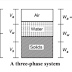Three phase diagram :

The phase diagram is a simple, it is a diagrammatic representation of a real soil. The phase diagram is also known as block diagram in soil mechanics. A soil mass consists of solid particles, water and air,  which are segregated and placed separately, known as  three-phase system.A three-phase system becomes a two-phase system when  the soil is absolutely dry like (solids + air) or when the soil is  fully saturated (solids + water).

In phase diagram, volumes are represented on the left  side, and weights are represented on the right side.

Volumetric Relationships

1. Void ratio (e): It is defined as the ratio of volume of  voids to volume of solids.

Limit: e > 0

For some soils, it may have a value even greater than unity.and the void ratio of coarse grained soils is smaller than that of a fine-grained soil.

2. Porosity (n): It is defined as the ratio of volume of  voids to the total volume.

Limit: 0 < n < 1

3.Degree of saturation (Sr): It is defined as the ratio of  volume of water to the volume of voids, in soil.

Limit: 0 ≤ S ≤ 100%

For dry soil, Vw = 0 ⇒ S = 0
For saturated soil, Vw = Vv ⇒ S = 100%

4.Percentage air voids (na): It is defined as the ratio of  volume of air to total volume, of soil.

Limit: 0 ≤ na  ≤ n

5.Air content (ac): It is defined as the ratio of volume of air to the volume of voids, in soil.

Limit: 0 ≤ ac  ≤ 100%

For dry soil, Va = Vv ⇒ ac  = 100%
For saturated soil, Va = 0 ⇒ ac  = 0

Three Phase system and Volumetric RelationshipsReviewed by Parag Pal on 10:30 PM Rating: 5